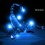Geometry prob

The problem: Let A be an outside point from the circle with the center O. Draw the secant ABC of the mentioned circle. The tangents at B and C got an intersection, named K. From K, we draw the perpendicular to AO. Name the intersection H. E and F are 2 intersections of KH and the circle with the center O. (E is between K and F). We name M for the intersection of KO and BC. Prove that:

1. There exists a circle that pass throught 4 points E, M, O and F
2. AE and AF are tangents of the circle with center O

Notes:

• I tried to translate this problem from my language to English. So If I use wrong grammar structure or wrong expression, please forgive me.
• This is not my homework. I like to look for and solve "hard" problems, by my view. This is one of those, and I can't find out the way to solve it.Note by Đức Việt Lê
6 years, 4 months ago

This discussion board is a place to discuss our Daily Challenges and the math and science related to those challenges. Explanations are more than just a solution — they should explain the steps and thinking strategies that you used to obtain the solution. Comments should further the discussion of math and science.

When posting on Brilliant:

• Use the emojis to react to an explanation, whether you're congratulating a job well done , or just really confused .
• Ask specific questions about the challenge or the steps in somebody's explanation. Well-posed questions can add a lot to the discussion, but posting "I don't understand!" doesn't help anyone.
• Try to contribute something new to the discussion, whether it is an extension, generalization or other idea related to the challenge.
• Stay on topic — we're all here to learn more about math and science, not to hear about your favorite get-rich-quick scheme or current world events.

MarkdownAppears as
*italics* or _italics_ italics
**bold** or __bold__ bold

- bulleted
- list

• bulleted
• list

1. numbered
2. list

1. numbered
2. list
Note: you must add a full line of space before and after lists for them to show up correctly
paragraph 1

paragraph 2

paragraph 1

paragraph 2

> This is a quote
This is a quote
# I indented these lines
# 4 spaces, and now they show
# up as a code block.

print "hello world"
# I indented these lines
# 4 spaces, and now they show
# up as a code block.

print "hello world"
MathAppears as
Remember to wrap math in $$...$$ or $...$ to ensure proper formatting.
2 \times 3 $2 \times 3$
2^{34} $2^{34}$
a_{i-1} $a_{i-1}$
\frac{2}{3} $\frac{2}{3}$
\sqrt{2} $\sqrt{2}$
\sum_{i=1}^3 $\sum_{i=1}^3$
\sin \theta $\sin \theta$
\boxed{123} $\boxed{123}$

Sort by:

This problem can be solved by the similarity relationships in a right triangle with the altitude of the hypotenuse. Here's a sketch of my solution: 1. By the power of a point theorem(or whatever you want to call it, it's basically simiarity), we get that KExKF=KB^2. Moreover, since ∠KBO=90° and BM⊥KO, we get KMxKO=KB^2=KExKF→O,M,E,F are concyclic. 2. We know that AE is tangent to circle O iff ∠OEA=90°. Since ∠KMA=∠KHA=90°, so K,M,H,A are concyclic. Thus OHxOA=OMxMK=OB^2=OE^2→By SAS similarity △EOH is similar to △AOE→∠OEA=∠EHO=90° and that proves AE is tangent to circle O. By analogy AF is also tangent to circle O.

- 6 years, 4 months ago

sorry for not following the formatting guidline :(

- 6 years, 4 months ago

Thanks a lot! I can understand the solution that you gave me. But I cannot figure it out :D

- 6 years, 4 months ago